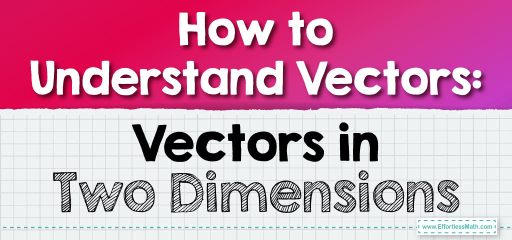# How to Understand Vectors: Vectors in Two Dimensions

A vector is a mathematical object that has both magnitude (or size) and direction. Unlike a scalar, which only has magnitude, a vector provides information about how much## Step-by-step Guide to Understand Vectors

Here is a step-by-step guide to understand vectors:

### Step 1: Understanding the Basics

• Definition: A vector is a mathematical representation of both magnitude and direction. Think of it as an arrow pointing from one point to another.
• Components: In two dimensions, a vector has two parts: the $$x$$-component and the $$y$$-component, representing horizontal and vertical directions, respectively.

### Step 2: Graphical Representation

• Axes Establishment: Start with a two-dimensional Cartesian plane. Label the horizontal axis as ‘$$x$$’ and the vertical axis as ‘$$y$$’.
• Drawing Vectors: Represent a vector as an arrow. The tail starts at the origin $$(0,0)$$ and the head (or tip) ends at the coordinate given by its $$x$$ and $$y$$ components.
• Length and Direction: The length of the arrow represents the magnitude of the vector. The direction in which it points gives, well, its direction.

### Step 3: Defining Vectors Algebraically

• Notation: Typically, vectors are represented by bold letters or letters with an arrow above, like $$v$$ or $$→v$$.
• Components: A vector in $$2D$$ can be written as $$(x,y)$$ or $$xi + yj$$, where $$x$$ and $$y$$ are scalars and $$i$$ and $$j$$ are unit vectors in the $$x$$ and $$y$$ directions.

### Step 4: Vector Operations

• Vector Addition: To add vectors, add their respective components. For vectors $$a = (x₁,y₁)$$ and $$b = (x₂,y₂)$$, the sum is $$a + b = (x₁+x₂, y₁+y₂)$$.
• Vector Subtraction: Similarly, subtract the components: $$a – b = (x₁-x₂, y₁-y₂)$$.
• Scalar Multiplication: Multiply each component of a vector by a scalar. For scalar $$k$$ and vector $$a = (x,y), ka = (kx, ky)$$.

### Step 5: Magnitude and Direction

• Magnitude (or length): Given a vector $$(x,y)$$, its magnitude is found by the Pythagorean theorem: $$|v| = \sqrt{(x² + y²)}$$.
• Direction: Using trigonometry, the angle $$θ$$ (from the positive $$x$$-axis) is given by $$θ = tan⁻¹(\frac{y}{x})$$.

### Step 6: Unit Vectors

• Definition: A unit vector has a magnitude of one and points in a specific direction.
• In $$2D$$: The primary unit vectors are $$i$$ (which points in the positive $$x$$-direction) and $$j$$ (which points in the positive $$y$$-direction).

### Step 7: Dot Product

• Definition: It’s a way to multiply vectors resulting in a scalar. For vectors $$a = (x₁,y₁)$$ and $$b = (x₂,y₂)$$, the dot product is given by $$a • b = x₁x₂ + y₁y₂$$.
• Orthogonal Vectors: If $$a • b = 0$$, then vectors $$a$$ and $$b$$ are orthogonal (or perpendicular).

### Step 8: Practical Applications

• Physics: Vectors are crucial in representing quantities like force, velocity, and acceleration.
• Computer Graphics: Used to describe positions, directions, and more, aiding in the creation of complex visualizations and animations.

### Step 9: Beyond the Basics

• Once comfortable with vectors in two dimensions, expand your knowledge to three-dimensional vectors. Dive deeper into vector calculus, and explore concepts like cross-products and curl.

By taking a sequential, layered approach to vectors, you’ll build a robust foundation that can support more advanced mathematical and applied concepts. Vectors, though simple in concept, play a fundamental role in various disciplines, from physics to engineering to computer science. Dive in, explore, and let vectors guide your path through the intricate tapestry of mathematics.

### Examples:

Show vector $$B=(4,2)$$ in the coordinate plane.

Solution:

1. Setting up the Coordinate Plane:
• Draw a set of perpendicular lines, the $$x$$-axis (horizontal) and the $$y$$-axis (vertical). These represent your coordinate plane.
• Mark intervals on each axis, similar to a grid, to denote units.
2. Plotting the Vector:
• Start at the origin, the point $$(0,0)$$.
• Move $$4$$ units to the right along the $$x$$-axis. This represents the $$x$$-component of the vector.
• From that point, move $$2$$ unit upwards. This represents the $$y$$-component of the vector.
• Draw an arrow starting from the origin to the point you’ve reached, which is $$(4,2)$$. The arrow represents vector $$B$$.

### What people say about "How to Understand Vectors: Vectors in Two Dimensions - Effortless Math: We Help Students Learn to LOVE Mathematics"?

No one replied yet.

X
30% OFF

Limited time only!

Save Over 30%

SAVE $5 It was$16.99 now it is \$11.99